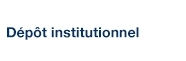# Dehn fillings of large hyperbolic 3-manifolds

Boyer, Steven; Gordon, C. McA. et Zhang, X. (2001). « Dehn fillings of large hyperbolic 3-manifolds ». Journal of Differential Geometry, 58(2), pp. 263-308.

Fichier(s) associé(s) à ce document :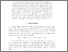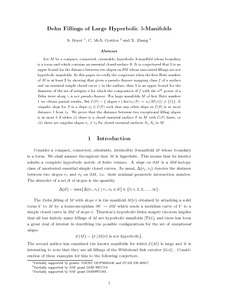Prévisualisation
PDF
Télécharger (343kB)

## Résumé

Let M be a compact, connected, orientable, hyperbolic 3-manifold whose boundary is a torus and which contains an essential closed surface S. It is conjectured that 5 is an upper bound for the distance between two slopes on ∂M whose associated fillings are not hyperbolic manifolds. In this paper we verify the conjecture when the first Betti number of M is at least 2 by showing that given a pseudo-Anosov mapping class f of a surface and an essential simple closed curve γ in the surface, then 5 is an upper bound for the diameter of the set of integers n for which the composition of f with the nth power of a Dehn twist along γ is not pseudo-Anosov. This sharpens an inequality of Albert Fathi. For large manifolds M of first Betti number 1 we obtain partial results. Set C(S) = {slopes r | ker(π1(S) → π1(M(r))) ≠ {1}}. A singular slope for S is a slope r0 ∈ C(S) such that any other slope in C(S) is at most distance 1 from r0. We prove that the distance between two exceptional filling slopes is at most 5 if either (i) there is a closed essential surface S in M with C(S) finite, or (ii) there are singular slopes r1 ≠ r2 for closed essential surfaces S1, S2 in M.

Type: Article de revue scientifique Dehn fillings, hyperbolic 3-manifold Faculté des sciences > Département de mathématiques Steven P. Boyer 26 avr. 2016 19:25 30 mai 2016 14:39 http://archipel.uqam.ca/id/eprint/8319

## Statistiques

Voir les statistiques sur cinq ans...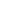RECHERCHER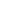PARCOURIR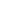LIBRE ACCÈS